### IMO Shortlist 1996 problem G3

Kvaliteta:
Avg: 4,0
Težina:
Avg: 7,0
Let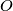$O$ be the circumcenter and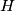$H$ the orthocenter of an acute-angled triangle$ABC$ such that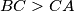$BC>CA$. Let$F$ be the foot of the altitude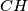$CH$ of triangle$ABC$. The perpendicular to the line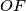$OF$ at the point$F$ intersects the line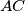$AC$ at$P$. Prove that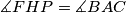$\measuredangle FHP=\measuredangle BAC$.
Izvor: Međunarodna matematička olimpijada, shortlist 1996